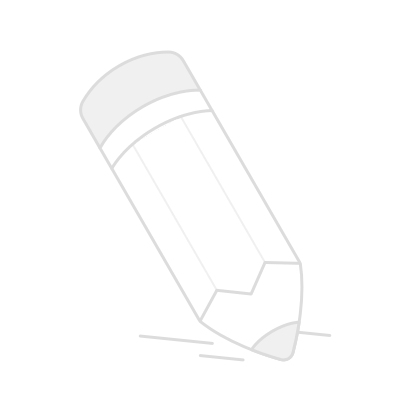# 3 数论3： 五个数的和的末尾数字

2010 = 1 ×2 × 3 × 5 × 67

x - a = 1,  x - b = 2,  x - c = 3, x - d = 5,  x - e = 67

abcde是五个不同的正整数，有无数种可能。取x 的最小值1 + 67 = 68，把68赋值给x

（68- 67）（68 - 66）（68 - 65）（68- 63）（68 - 1）

b是唯一的偶数，让b = 66 + 2k

x - b = 2

x = 68 + 2k

x-ax-e, 一共加了 2k * 5 = 10k，求和时末位数不变。

（100- 99）（100 - 98）（100 - 97）（100- 95）（100 - 33）

a+b+c+d+e = 5x - [x-a+x-b+x-c+x-d+x-e]

x-a+x-b+x-c+x-d+x-e）的和是1+2+3+5+67，末位数字：8

5x的末位数。
abcde中有且只有一个是偶数，

∴其他4个数都是奇数

x-a,  x-b,  x-c,  x-d,  x-e 有一项是2

偶数 - 偶数 = 偶数，偶数 奇数 = 奇数

∴有且只有一项式子为偶数

x只能为偶数， 5x的末尾数字为0，即为10 – 8 = 2

math001.com/iq  数论 → 第7题

## 用户评论(0)展示条数：20条20条50条100条